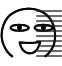### 学习笔记 - 学Python的第2天

• 1.7k阅读
• 2022年05月08日
• 0评论
/ / 正文

（不要在意发布文章的时间，因为并不是学完就发的## 运算符

### 算术运算符

+两个对象相加 a + b 输出结果 30
---得到负数或是一个数减去另一个数 a - b 输出结果 -10
*两个数相乘或是返回一个被重复若干次的字符串 a * b 输出结果 200
/b / a 输出结果 2
//取整除返回商的整数部分 9//2 输出结果 4 , 9.0//2.0 输出结果 4.0
%取余返回除法的余数 b % a 输出结果 0
**指数a**b 为10的20次方， 输出结果 100000000000000000000
()小括号提高运算优先级，比如: (1+2) * 3
# 加
a = 10 + 20
print(a) #30

# 减
a = 20 - 10
print(a) #10

# 乘
a = 10 * 10
print(a) #100

# 除
a = 20 / 10
print(a) #2.0

# // 取整数位
a = 9//2
print(a) #4

# % 取余数位
a = 9%2
print(a) #1

# 提高运算优先级
# 不使用()提升优先级
a = 10+10*2
print(a) #30
# 使用()提升优先级
a = (10+10)*2
print(a) #40

### 赋值运算符

=赋值运算符把 = 号右边的结果 赋给 左边的变量，如 num = 1 + 2 * 3，结果num的值为7
# 单个变量赋值
num = 10
print(num) #10

# 多个变量赋值
num1, f1, str1 = 100, 3.14, "hello"

# 同时为多个变量赋值
a = b = 4

# 语法错误，不能在小括号里写赋值语句
# a = (b = 4)

### 复合赋值运算符

+=加法赋值运算符c += a 等效于 c = c + a
-=减法赋值运算符c -= a 等效于 c = c - a
*=乘法赋值运算符c *= a 等效于 c = c * a
/=除法赋值运算符c /= a 等效于 c = c / a
%=取模赋值运算符c %= a 等效于 c = c % a
**=幂赋值运算符c **= a 等效于 c = c ** a
//=取整除赋值运算符c //= a 等效于 c = c // a
# 示例：+=
a = 100
a += 1  # 相当于执行 a = a + 1
print(a) #101

# 示例：*=
a = 100
a *= 2  # 相当于执行 a = a * 2
print(a) #200

# 示例：*=，运算时，符号右侧的表达式先计算出结果，再与左边变量的值运算
a = 100
a *= 1 + 2  # 相当于执行 a = a * (1+2)
print(a) #300

## 数据类型转换

### 常用的数据类型转换

int(x [,base ])将x转换为一个整数
float(x )将x转换为一个浮点数
complex(real [,imag ])创建一个复数，real为实部，imag为虚部
str(x )将对象 x 转换为字符串
repr(x )将对象 x 转换为表达式字符串
eval(str )用来计算在字符串中的有效Python表达式,并返回一个对象
tuple(s )将序列 s 转换为一个元组
list(s )将序列 s 转换为一个列表
chr(x )将一个整数转换为一个Unicode字符
ord(x )将一个字符转换为它的ASCII整数值
hex(x )将一个整数转换为一个十六进制字符串
oct(x )将一个整数转换为一个八进制字符串
bin(x )将一个整数转换为一个二进制字符串
# 不想写示例了。。。